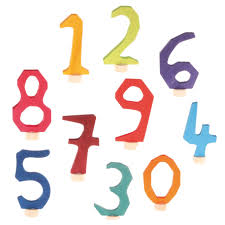# Numbers:Same Numbers Ending with 5.

75 x 75 = 5625

85 x 85 = 7225

105 x 105 = 11025

First multiyply last numbers of both figures.
so 5 x 5 = 25, then Next to 7 digit is 8,
so you can multiyply 7 * 8 = 56, then 5625.

Like wise you can observe three examples for your reference.
This sequence will be useful, only in case of same numbers
should be there in both sides.

Wishing you all the best,
http://www.seeyourneeds.in DONATE AS YOU LIKE